Best for live in-class or video conferencing lessonsStart teacher-led lesson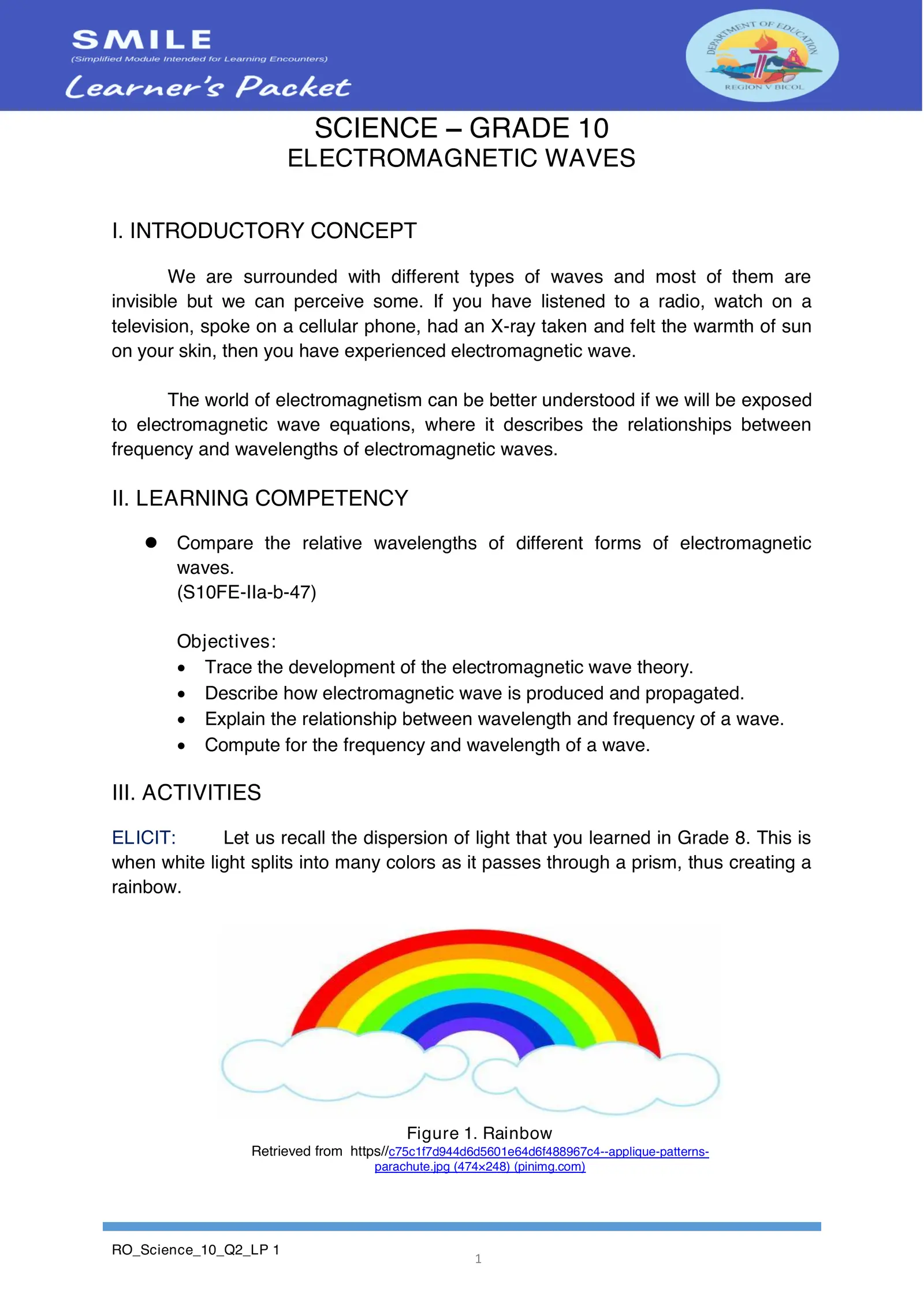SCIENCE – GRADE 10 ELECTROMAGNETIC WAVES I. INTRODUCTORY CONCEPT We are surrounded with different types of waves and most of them are invisible but we can perceive some. If you have listened to a radio, watch on a television, spoke on a cellular phone, had an X-ray taken and felt the warmth of sun on your skin, then you have experienced electromagnetic wave. The world of electromagnetism can be better understood if we will be exposed to electromagnetic wave equations, where it describes the relationships between frequency and wavelengths of electromagnetic waves. II. LEARNING COMPETENCY  Compare the relative wavelengths of different forms of electromagnetic waves. (S10FE-IIa-b-47) Objectives:  Trace the development of the electromagnetic wave theory.  Describe how electromagnetic wave is produced and propagated.  Explain the relationship between wavelength and frequency of a wave.  Compute for the frequency and wavelength of a wave. III. ACTIVITIES ELICIT: Let us recall the dispersion of light that you learned in Grade 8. This is when white light splits into many colors as it passes through a prism, thus creating a rainbow. Figure 1. Rainbow Retrieved from https//c75c1f7d944d6d5601e64d6f488967c4--applique-patterns- parachute.jpg (474×248) (pinimg.com) RO_Science_10_Q2_LP 1 1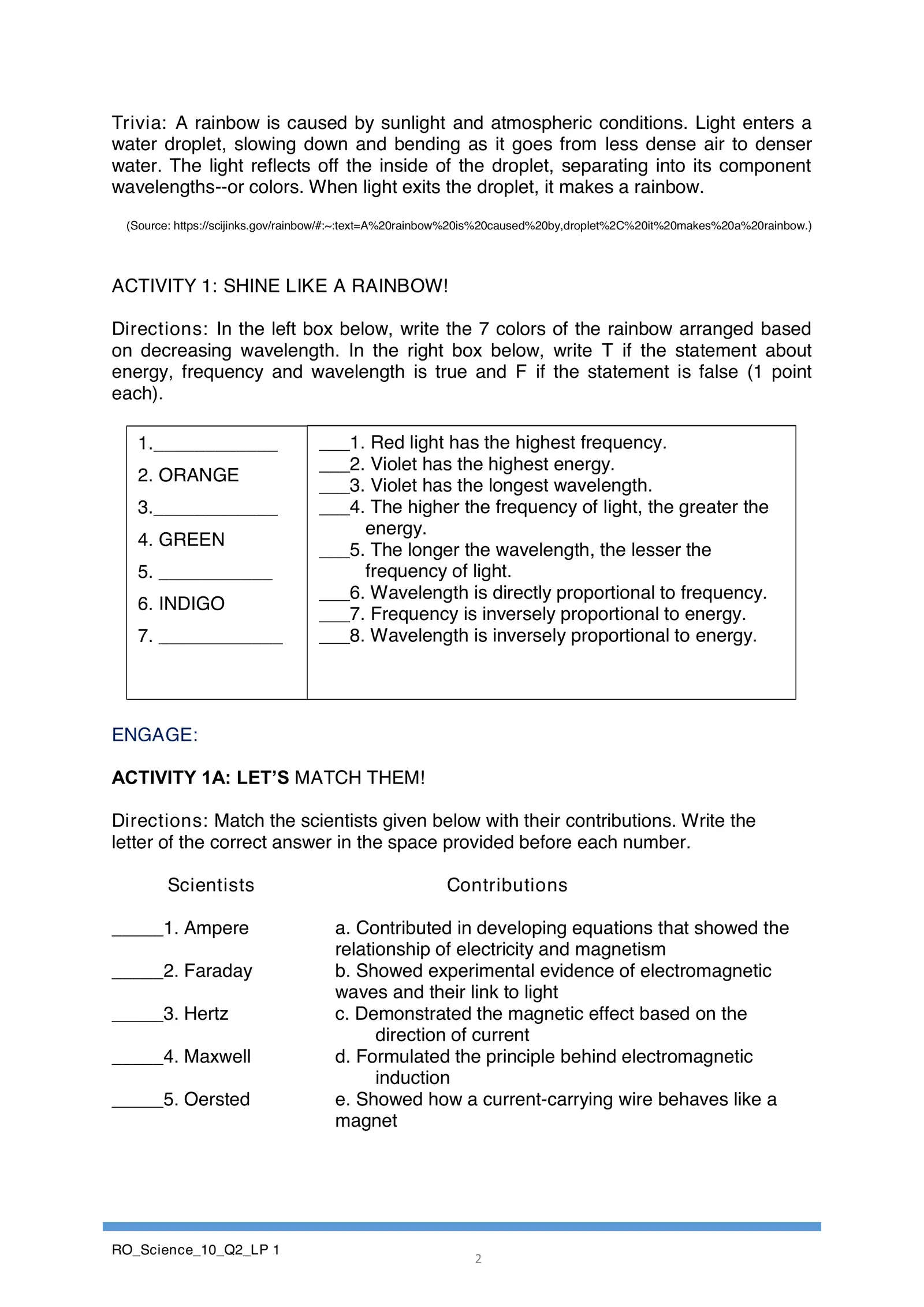Trivia: A rainbow is caused by sunlight and atmospheric conditions. Light enters a water droplet, slowing down and bending as it goes from less dense air to denser water. The light reflects off the inside of the droplet, separating into its component wavelengths--or colors. When light exits the droplet, it makes a rainbow. (Source: https://scijinks.gov/rainbow/#:~:text=A%20rainbow%20is%20caused%20by,droplet%2C%20it%20makes%20a%20rainbow.) ACTIVITY 1: SHINE LIKE A RAINBOW! Directions: In the left box below, write the 7 colors of the rainbow arranged based on decreasing wavelength. In the right box below, write T if the statement about energy, frequency and wavelength is true and F if the statement is false (1 point each). 1.____________ ___1. Red light has the highest frequency. ___2. Violet has the highest energy. 2. ORANGE ___3. Violet has the longest wavelength. 3.____________ ___4. The higher the frequency of light, the greater the energy. 4. GREEN ___5. The longer the wavelength, the lesser the 5. ___________ frequency of light. ___6. Wavelength is directly proportional to frequency. 6. INDIGO ___7. Frequency is inversely proportional to energy. 7. ____________ ___8. Wavelength is inversely proportional to energy. ENGAGE: ACTIVITY 1A: LET’S MATCH THEM! Directions: Match the scientists given below with their contributions. Write the letter of the correct answer in the space provided before each number. Scientists Contributions _____1. Ampere a. Contributed in developing equations that showed the relationship of electricity and magnetism _____2. Faraday b. Showed experimental evidence of electromagnetic waves and their link to light _____3. Hertz c. Demonstrated the magnetic effect based on the direction of current _____4. Maxwell d. Formulated the principle behind electromagnetic induction _____5. Oersted e. Showed how a current-carrying wire behaves like a magnet RO_Science_10_Q2_LP 1 2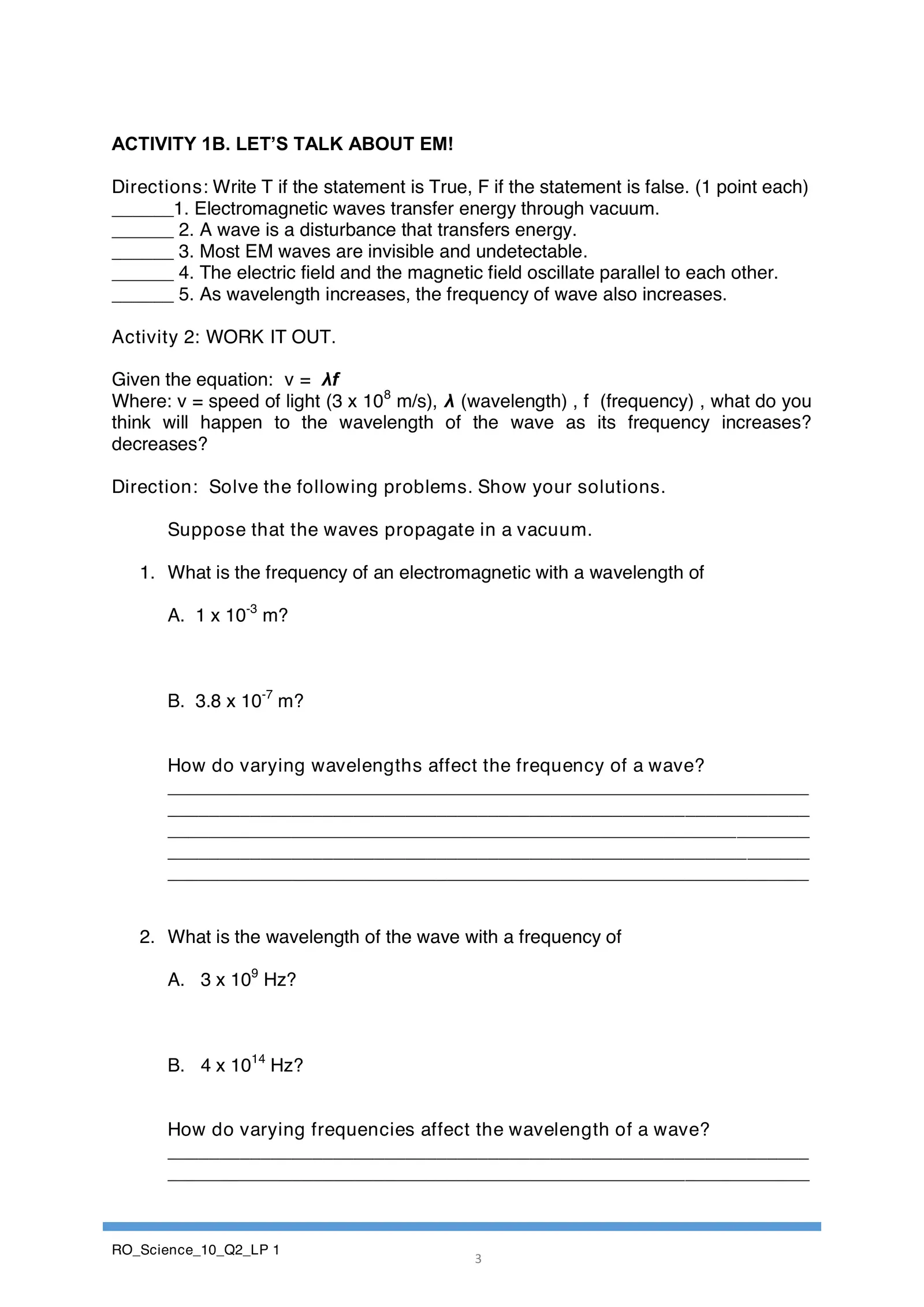ACTIVITY 1B. LET’S TALK ABOUT EM! Directions: Write T if the statement is True, F if the statement is false. (1 point each) ______1. Electromagnetic waves transfer energy through vacuum. ______ 2. A wave is a disturbance that transfers energy. ______ 3. Most EM waves are invisible and undetectable. ______ 4. The electric field and the magnetic field oscillate parallel to each other. ______ 5. As wavelength increases, the frequency of wave also increases. Activity 2: WORK IT OUT. Given the equation: v = λf Where: v = speed of light (3 x 108 m/s), λ (wavelength) , f (frequency) , what do you think will happen to the wavelength of the wave as its frequency increases? decreases? Direction: Solve the following problems. Show your solutions. Suppose that the waves propagate in a vacuum. 1. What is the frequency of an electromagnetic with a wavelength of A. 1 x 10-3 m? B. 3.8 x 10-7 m? How do varying wavelengths affect the frequency of a wave? ______________________________________________________________ ______________________________________________________________ ______________________________________________________________ ______________________________________________________________ ______________________________________________________________ 2. What is the wavelength of the wave with a frequency of A. 3 x 109 Hz? B. 4 x 1014 Hz? How do varying frequencies affect the wavelength of a wave? ______________________________________________________________ ______________________________________________________________ RO_Science_10_Q2_LP 1 3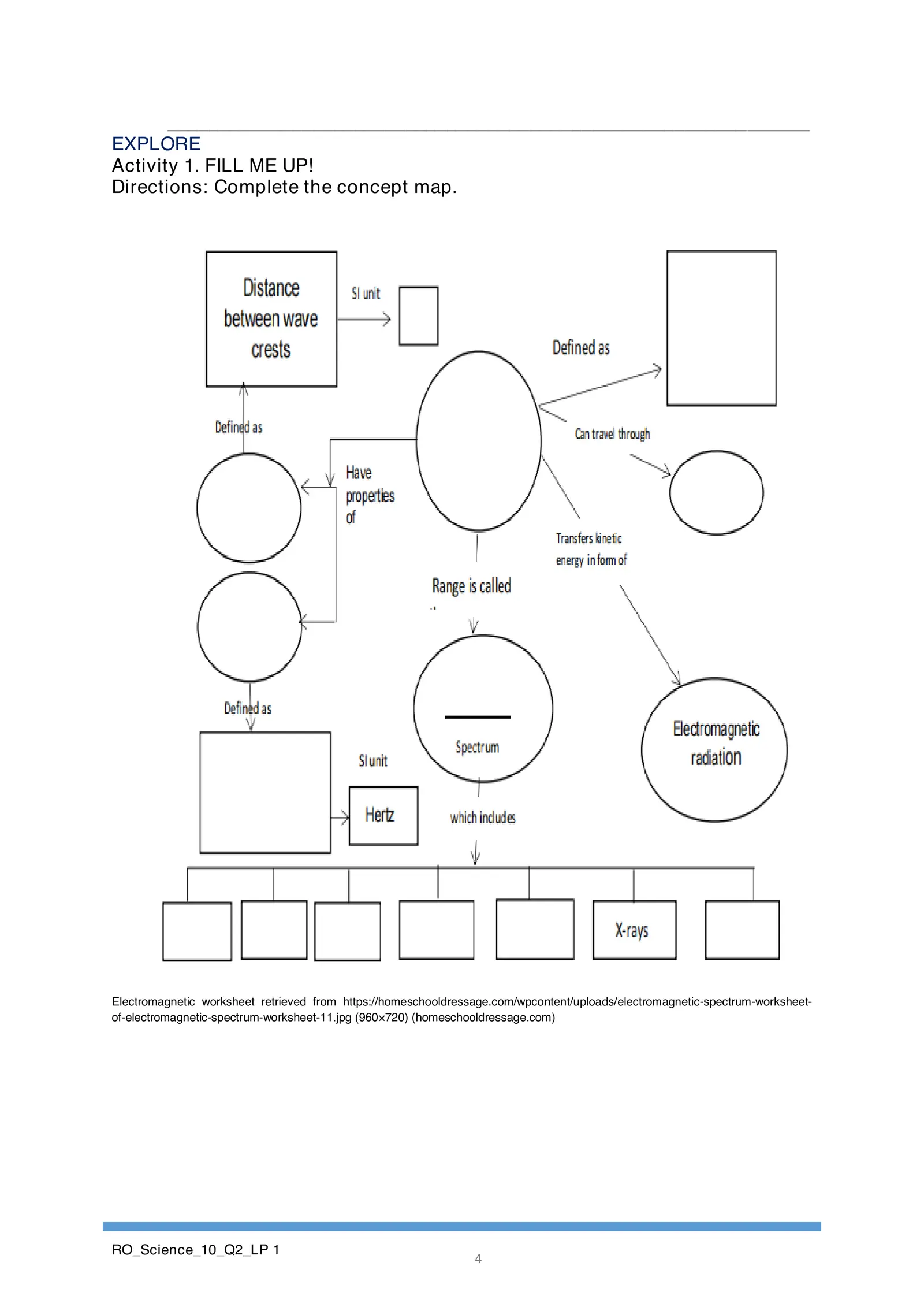______________________________________________________________ EXPLORE Activity 1. FILL ME UP! Directions: Complete the concept map. Electromagnetic worksheet retrieved from https://homeschooldressage.com/wpcontent/uploads/electromagnetic-spectrum-worksheet- of-electromagnetic-spectrum-worksheet-11.jpg (960×720) (homeschooldressage.com) RO_Science_10_Q2_LP 1 4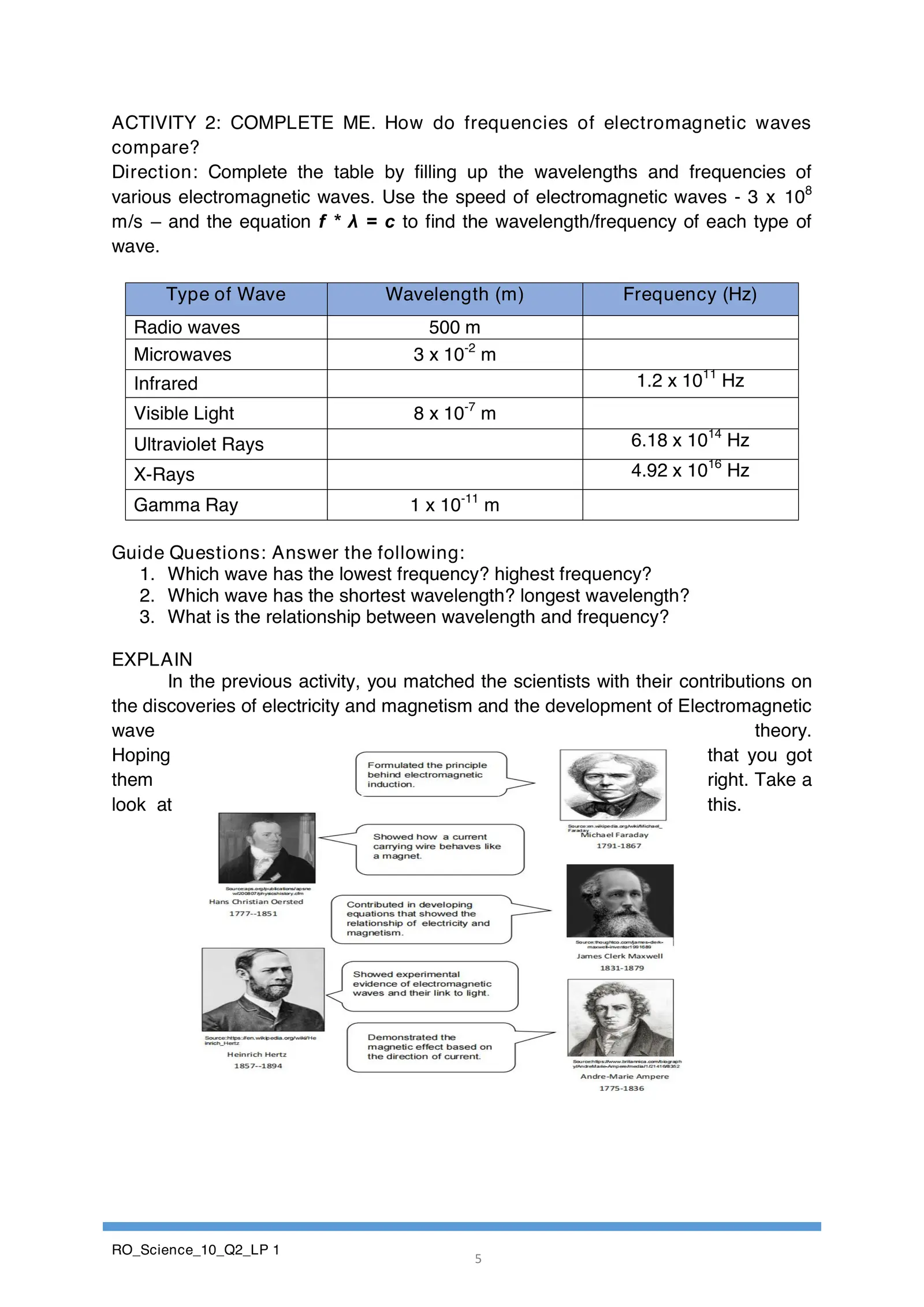ACTIVITY 2: COMPLETE ME. How do frequencies of electromagnetic waves compare? Direction: Complete the table by filling up the wavelengths and frequencies of various electromagnetic waves. Use the speed of electromagnetic waves - 3 x 108 m/s – and the equation f * λ = c to find the wavelength/frequency of each type of wave. Type of Wave Wavelength (m) Frequency (Hz) Radio waves 500 m Microwaves 3 x 10-2 m Infrared 1.2 x 1011 Hz Visible Light 8 x 10-7 m Ultraviolet Rays 6.18 x 1014 Hz X-Rays 4.92 x 1016 Hz Gamma Ray 1 x 10-11 m Guide Questions: Answer the following: 1. Which wave has the lowest frequency? highest frequency? 2. Which wave has the shortest wavelength? longest wavelength? 3. What is the relationship between wavelength and frequency? EXPLAIN In the previous activity, you matched the scientists with their contributions on the discoveries of electricity and magnetism and the development of Electromagnetic wave theory. Hoping that you got them right. Take a look at this. RO_Science_10_Q2_LP 1 5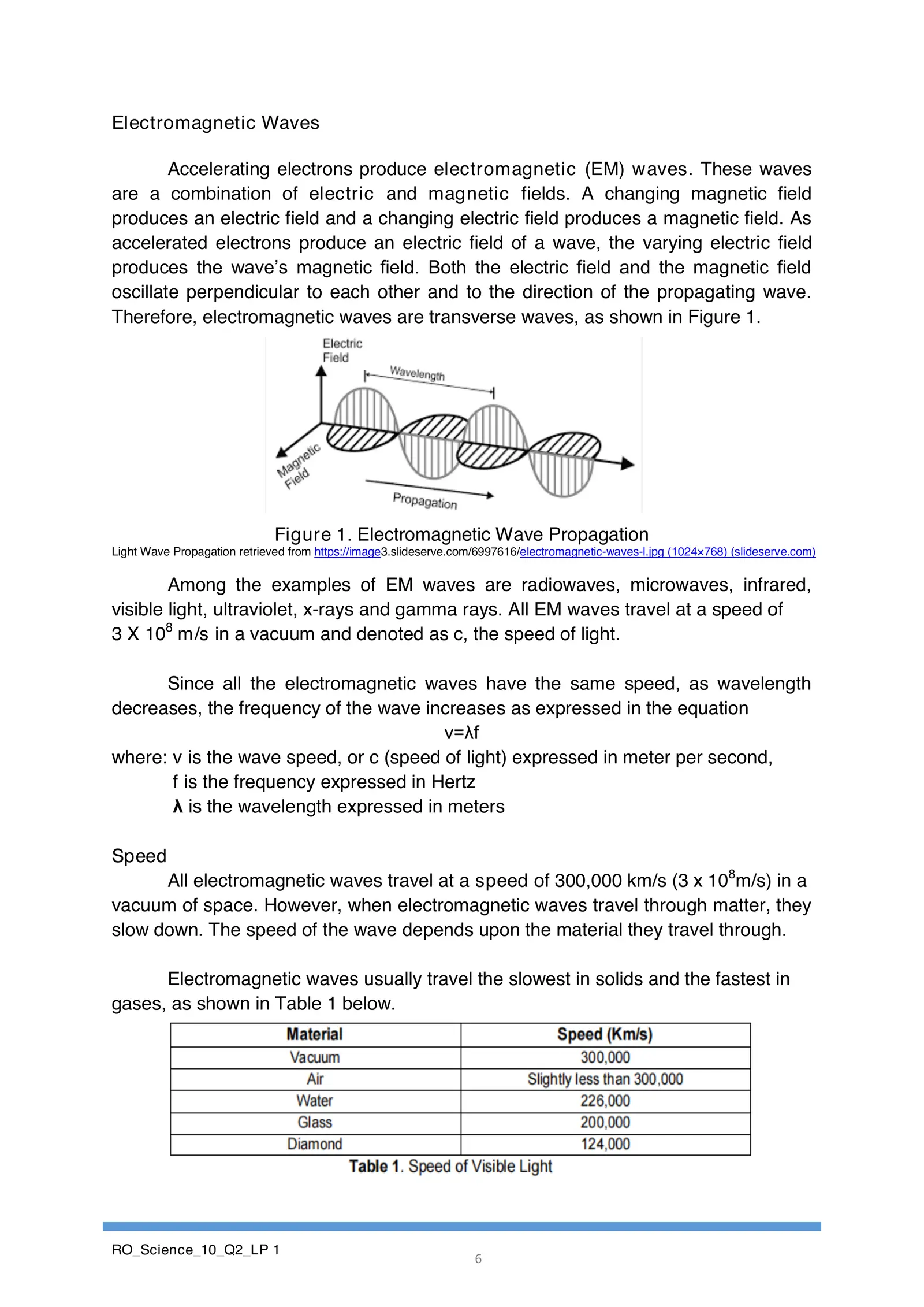Electromagnetic Waves Accelerating electrons produce electromagnetic (EM) waves. These waves are a combination of electric and magnetic fields. A changing magnetic field produces an electric field and a changing electric field produces a magnetic field. As accelerated electrons produce an electric field of a wave, the varying electric field produces the wave’s magnetic field. Both the electric field and the magnetic field oscillate perpendicular to each other and to the direction of the propagating wave. Therefore, electromagnetic waves are transverse waves, as shown in Figure 1. Figure 1. Electromagnetic Wave Propagation Light Wave Propagation retrieved from https://image3.slideserve.com/6997616/electromagnetic-waves-l.jpg (1024×768) (slideserve.com) Among the examples of EM waves are radiowaves, microwaves, infrared, visible light, ultraviolet, x-rays and gamma rays. All EM waves travel at a speed of 3 X 108 m/s in a vacuum and denoted as c, the speed of light. Since all the electromagnetic waves have the same speed, as wavelength decreases, the frequency of the wave increases as expressed in the equation v=λf where: v is the wave speed, or c (speed of light) expressed in meter per second, f is the frequency expressed in Hertz λ is the wavelength expressed in meters Speed All electromagnetic waves travel at a speed of 300,000 km/s (3 x 108m/s) in a vacuum of space. However, when electromagnetic waves travel through matter, they slow down. The speed of the wave depends upon the material they travel through. Electromagnetic waves usually travel the slowest in solids and the fastest in gases, as shown in Table 1 below. RO_Science_10_Q2_LP 1 6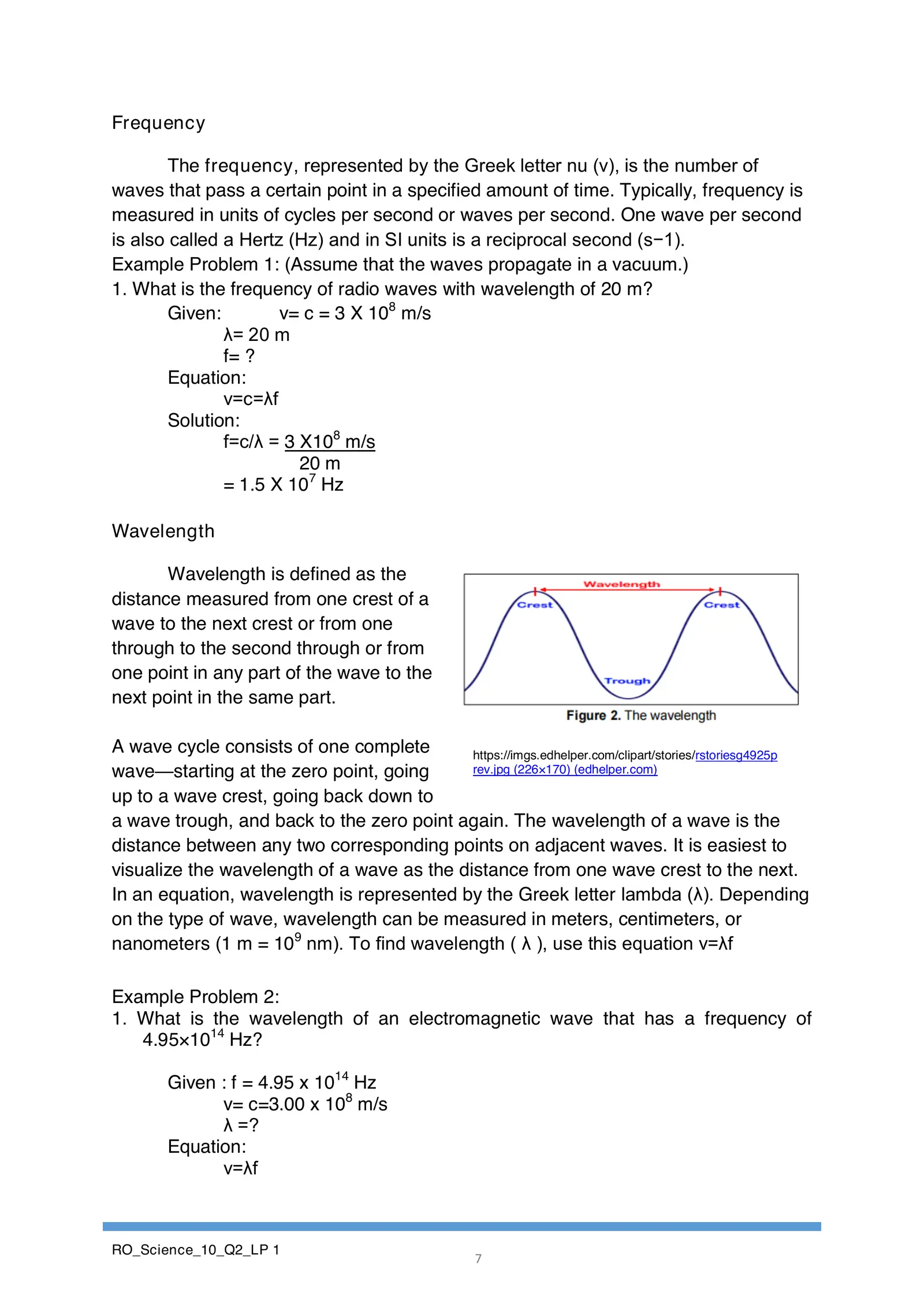Frequency The frequency, represented by the Greek letter nu (ν), is the number of waves that pass a certain point in a specified amount of time. Typically, frequency is measured in units of cycles per second or waves per second. One wave per second is also called a Hertz (Hz) and in SI units is a reciprocal second (s−1). Example Problem 1: (Assume that the waves propagate in a vacuum.) 1. What is the frequency of radio waves with wavelength of 20 m? Given: v= c = 3 X 108 m/s λ= 20 m f= ? Equation: v=c=λf Solution: f=c/λ = 3 X108 m/s 20 m = 1.5 X 107 Hz Wavelength Wavelength is defined as the distance measured from one crest of a wave to the next crest or from one through to the second through or from one point in any part of the wave to the next point in the same part. A wave cycle consists of one complete https://imgs.edhelper.com/clipart/stories/rstoriesg4925p wave—starting at the zero point, going rev.jpg (226×170) (edhelper.com) up to a wave crest, going back down to a wave trough, and back to the zero point again. The wavelength of a wave is the distance between any two corresponding points on adjacent waves. It is easiest to visualize the wavelength of a wave as the distance from one wave crest to the next. In an equation, wavelength is represented by the Greek letter lambda (λ). Depending on the type of wave, wavelength can be measured in meters, centimeters, or nanometers (1 m = 109 nm). To find wavelength ( λ ), use this equation v=λf Example Problem 2: 1. What is the wavelength of an electromagnetic wave that has a frequency of 4.95×1014 Hz? Given : f = 4.95 x 1014 Hz v= c=3.00 x 108 m/s λ =? Equation: v=λf RO_Science_10_Q2_LP 1 7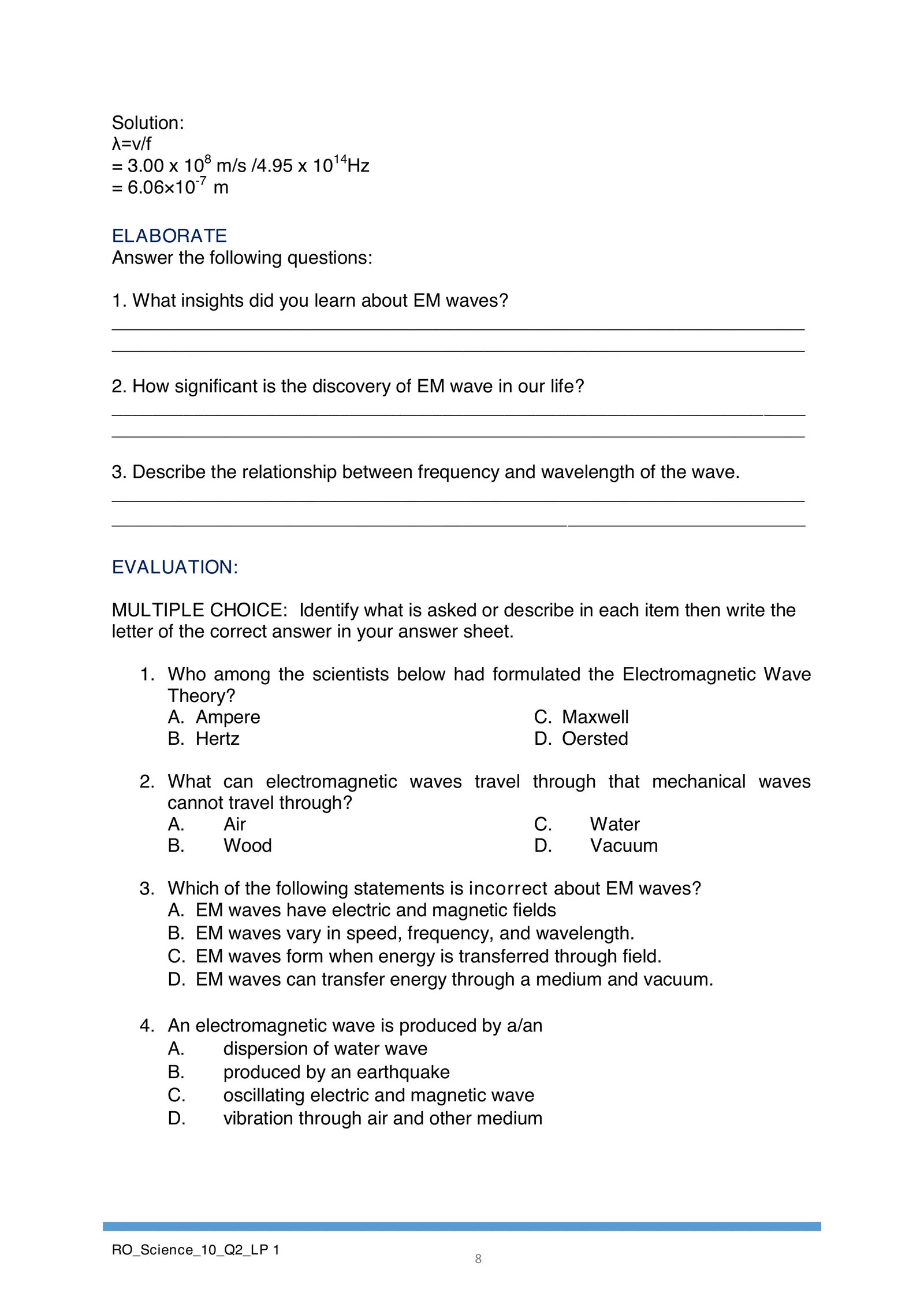Solution: λ=v/f = 3.00 x 108 m/s /4.95 x 1014Hz = 6.06×10-7 m ELABORATE Answer the following questions: 1. What insights did you learn about EM waves? ___________________________________________________________________ ___________________________________________________________________ 2. How significant is the discovery of EM wave in our life? ___________________________________________________________________ ___________________________________________________________________ 3. Describe the relationship between frequency and wavelength of the wave. ___________________________________________________________________ ___________________________________________________________________ EVALUATION: MULTIPLE CHOICE: Identify what is asked or describe in each item then write the letter of the correct answer in your answer sheet. 1. Who among the scientists below had formulated the Electromagnetic Wave Theory? A. Ampere C. Maxwell B. Hertz D. Oersted 2. What can electromagnetic waves travel through that mechanical waves cannot travel through? A. Air C. Water B. Wood D. Vacuum 3. Which of the following statements is incorrect about EM waves? A. EM waves have electric and magnetic fields B. EM waves vary in speed, frequency, and wavelength. C. EM waves form when energy is transferred through field. D. EM waves can transfer energy through a medium and vacuum. 4. An electromagnetic wave is produced by a/an A. dispersion of water wave B. produced by an earthquake C. oscillating electric and magnetic wave D. vibration through air and other medium RO_Science_10_Q2_LP 1 8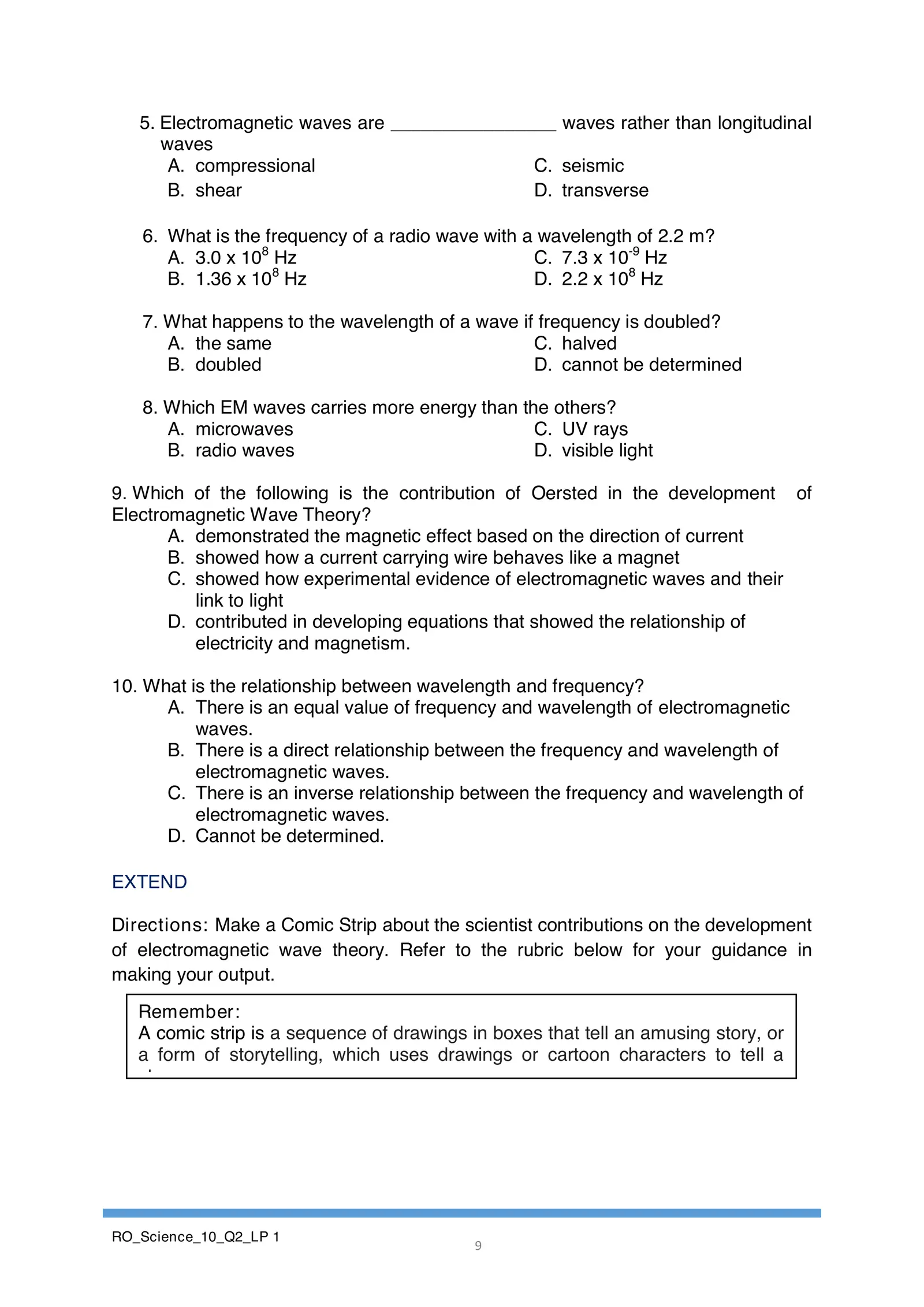5. Electromagnetic waves are ________________ waves rather than longitudinal waves A. compressional C. seismic B. shear D. transverse 6. What is the frequency of a radio wave with a wavelength of 2.2 m? A. 3.0 x 108 Hz C. 7.3 x 10-9 Hz 8 B. 1.36 x 10 Hz D. 2.2 x 108 Hz 7. What happens to the wavelength of a wave if frequency is doubled? A. the same C. halved B. doubled D. cannot be determined 8. Which EM waves carries more energy than the others? A. microwaves C. UV rays B. radio waves D. visible light 9. Which of the following is the contribution of Oersted in the development of Electromagnetic Wave Theory? A. demonstrated the magnetic effect based on the direction of current B. showed how a current carrying wire behaves like a magnet C. showed how experimental evidence of electromagnetic waves and their link to light D. contributed in developing equations that showed the relationship of electricity and magnetism. 10. What is the relationship between wavelength and frequency? A. There is an equal value of frequency and wavelength of electromagnetic waves. B. There is a direct relationship between the frequency and wavelength of electromagnetic waves. C. There is an inverse relationship between the frequency and wavelength of electromagnetic waves. D. Cannot be determined. EXTEND Directions: Make a Comic Strip about the scientist contributions on the development of electromagnetic wave theory. Refer to the rubric below for your guidance in making your output. Remember: A comic strip is a sequence of drawings in boxes that tell an amusing story, or a form of storytelling, which uses drawings or cartoon characters to tell a story. RO_Science_10_Q2_LP 1 9

Hold and speak
Watch the video below and answer the questions that follow: